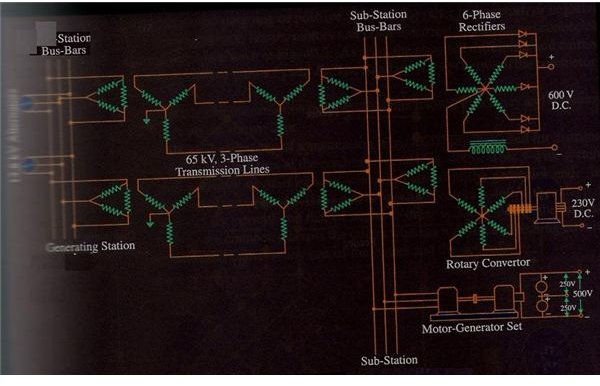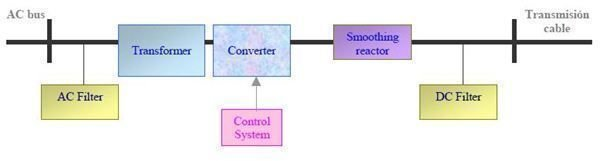High voltage DC power supplies – Learn how DC is transmitted

Introduction

After taking a look at the basics of electric power transmission and some facts of HVDC transmission, it is now time to learn some more facts about high voltage DC power supplies. Before that, just take a look at a typical system used to get DC power from an AC arrangement. The adjacent figure shows a simplified view of the overall power system from generation to transmission and final distribution. As you can see the various components of such a system are as follows.Power House – this is the point where power is generated from various sources which could be say hydroelectric power, thermal power and so on. The given scenario shows two generators running in parallel and feeding the bus bars.

Bus Bars – there are two sets of bus bars in the diagram. The first one is located at the generation site and the second one at the sub-station site.

Transformers – there are several transformers which step up the voltage at the generation point and then for lowering this voltage at the sub-station.

The final delivery mechanism varies with the load requirements and shown in the diagram are three ways to convert this AC power to DC power. Each of these devices gives a different output which is used to supply electricity to different consumers such as domestic, industrial and so forth.

So you can see that in the above case the transmission is via AC supply but the distribution is by DC supply.

HVDC System

Whereas the system described above is not a pure HVDC system because it does not use DC for transmission and only for distribution, there are systems being developed which use DC for transmission as well. Given below is the schematic diagram for such a system.As you can see from the diagram which is self explanatory and clearly labelled, the generated AC power is filtered and passed through the transformer for stepping up to appropriate level. After that it is converted to DC using a combination of thyristors or IGBT valves and again passed through filters before it is finally handed over to the transmission section.

Monopolar, Bipolar & 3 Wire Systems

The power from the substation can be transmitted using a single wire, two wires or even three wires. Of course the first two arrangements are normally used for transmission while the third is mainly used in distribution. We will take a brief look at all these three modes.

1. A monopolar transmission is carried out with the help of a single conductor. The return path is via the ground but due to its disadvantages many countries do not allow monopolar transmission of DC power
2. The bipolar is two wires, where one acts as the positive while other is the negative or return wire. The loads are connected in parallel across these two lines.
3. The 3 wire system has the highest efficiency of all the three systems and is mainly used for distribution from sub-station to actual consumers. It consists of two outer wires and a neutral wire. It has the advantage that the voltage is divided between the two sets formed by these three wires. So suppose the voltage between one set is 220 volts that can be used for domestic purposes by using the neutral and one outer wire; while the voltage for 440 volts can be achieved by making use of both outer wires and used for industry purposes.

At the consumer end we have feeders which refer to the lines from the sub-station till the distributor. The distributor distributes the power to the actual consumers via a network of sub-distributors and service main lines.

Image Credits

Electrical Technology by Dr Theraja